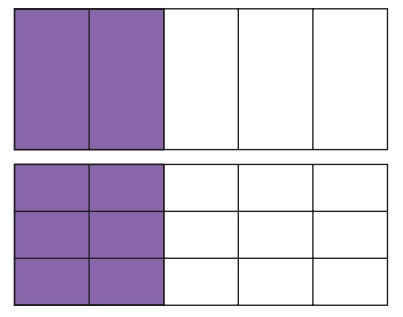Home | | Maths 5th Std | Equivalent fractions

# Equivalent fractions

If one chappathi is divided equally between two people then, each one will get half a chappathi. The fraction half, is written as 1/2. Here, 1 is the numerator and 2 is the denominator.

Equivalent fractions

If one chappathi is divided equally between two people then, each one will get half a chappathi. The fraction half, is written as 1/2. Here, 1 is the numerator and 2 is the denominator.The same chappathi is divided into four equal parts. Two parts were given to each person. This is shown as 2/4. Here 2 is the numerator and 4 is the denominator.

It means that the value of two fractions is the same. That is, 1/2 = 2/4

Such fractions of equal value are called equivalent fractions.

Obtaining equivalent fractionsTwo of the five equal parts in the figure are coloured. The coloured part is 2/5 of the whole figure.

When two lines are drawn horizontally in the same figure, the figure gets divided into 15 equal parts. So, the fraction that shows the coloured part now has changed. Now, the fraction of the coloured part is 6/15 but coloured size is the same.

Two figures that have the same size are called equivalent fractions.

Therefore, we see that 2/5 = 6/15

When the numerator and denominator of a fraction are multiplied by the same non-zero number, we get a fraction that is equivalent to the given fraction.

Example 6.4

Find a fraction with denominator 20 which is equivalent to 2/5 .

Solution

2/5 =/20 We must find the right number for the box.

Here, 4 times the denominator 5 is 20, so multiply the numerator also by 4

Therefore,2/5 = 2×4 / 5×4 = 8/20

Hence, the fraction 8/20 is equivalent to 2/5.

Example 6.5

Find a fraction equivalent to 8/20 with denominator 5.

Solution

8/20 =/5. We must find the number for the box.

In both the fractions denominator is given, that is 20 and 5.

Here, we get 5 in one of the fractions if we divide 20 (denominator of the other fraction) by 4.

So, we can get the required number (in box), if we divide 8 by 4.

That is 8 ÷ 4 = 2

Therefore 8/20 = 8÷4 / 20÷4 = 2/5Thus, the fraction 2/5 is equivalent to the fraction 8/20.

Tags : Fractions | Term 3 Chapter 6 | 5th Maths , 5th Maths : Term 3 Unit 6 : Fractions
Study Material, Lecturing Notes, Assignment, Reference, Wiki description explanation, brief detail
5th Maths : Term 3 Unit 6 : Fractions : Equivalent fractions | Fractions | Term 3 Chapter 6 | 5th Maths